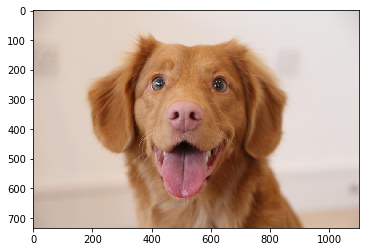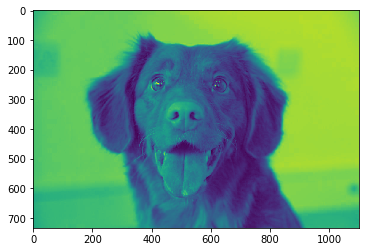## Introduction to NumPy

NumPy is one of the two most important libraries in Python for data science, along with Pandas. NumPy is a crucial library for effectively loading, storing, and manipulating in-memory data in Python, all of which will be at the heart of what you do with data science in Python.

Datasets come from a huge range of sources and in a wide range of formats, such as text documents, images, sound clips, numerical measurements, and nearly anything else. Despite this variety, however, the start of data science is to think of all data fundamentally as arrays of numbers.

For example, the words in documents can be represented as the numbers that encode letters in computers or even the frequency of particular words in a collection of documents. Digital images can be thought of as two-dimensional arrays of numbers representing pixel brightness or color. Sound files can be represented as one-dimensional arrays of frequency versus time. However, no matter what form our data takes, in order to analyze it, our first step will be to transform it into arrays of numbers—which is where NumPy comes in (and pandas down the road).

NumPy is short for Numerical Python, and it provides an efficient means of storing and operating on dense data buffers in Python. Array-oriented computing in Python goes back to 1995 with the Numeric library. Scientific programming in Python took off over the next 10 years, but the collections of libraries splintered. The NumPy project began in 2005 as a means of bringing the Numeric and NumArray projects together around a single array-based framework.

Some examples in this section are drawn from the Python Data Science Handbook by Jake VanderPlas (content available on GitHub) and Python for Data Analysis by Wes McKinney. Text from the Python Data Science Handbook is released under the CC-BY-NC-ND license; code is released under the MIT license.

Let's get started exploring NumPy! Our first step will be to import NumPy using `np` as an alias:

In :
``import jovian``
In :
``import numpy as np     #import the numpy library``
In :
``````import matplotlib.pyplot as plt       #importing matplotlibs as plt    Read the documentations
%matplotlib inline
``````
In :
``from PIL import Image      Import python imaging library to read the image using ``
In :
``pic=Image.open('puppy.jpg') #using the library upload the image"``
In :
``````pic

``````
Out:In :
``type(pic)   #now this is in the  format of jpg ``
Out:
``PIL.JpegImagePlugin.JpegImageFile``
In :
``````#now we have to convert it in the array format
pic_arr=np.array(pic)
``````
In :
``type(pic_arr)    #its in the array``
Out:
``numpy.ndarray``
In :
``pic_arr.shape   # 3 is the color channels``
Out:
``(734, 1100, 3)``
In :
``plt.imshow(pic_arr)``
Out:
``<matplotlib.image.AxesImage at 0x16377738a20>``In :
``````#lests make the copy of it

``````
In :
``pic_red=pic_arr.copy()``
In :
``pic_red.shape``
Out:
``(734, 1100, 3)``
In :
``plt.imshow(pic_red[:,:,2])``
Out:
``<matplotlib.image.AxesImage at 0x163778f6908>``In [ ]:
``jovian.commit()``
```[jovian] Saving notebook.. ```
In [ ]:
`` ``